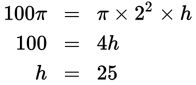# SAT Math Multiple Choice Question 982: Answer and Explanation

### Test Information

Question: 982

2. If a right cylinder with a radius of 2 cm has a volume of 100π cm3, what is the height, in centimeters, of the cylinder?

• A. 20
• B. 25
• C. 40
• D. 50

Explanation:

B

Difficulty: Easy

Category: Additional Topics in Math / Geometry

Strategic Advice: Use the formula for finding the volume of a cylinder to determine the missing dimension. On Test Day, don't forget that the formulas are provided for you on the reference page.

Getting to the Answer: The volume of a right cylinder is given by the formula V = πr2h. Plug the radius (2) and the volume (100π) into the formula, and then solve for the height:The height of the cylinder is 25 cm, which is (B).